# Physics - Reflection and Refraction

## Introduction

• The natural/artificial agent that kindles sight and makes things visible is known as light.• Light appears to travel in straight lines.

## Reflection of light

• The throwing back by a body or surface of light without absorbing it, is known as reflection of light.

• A highly polished surface, such as a mirror or other smooth and plane surface, reflects most of the light falling on it.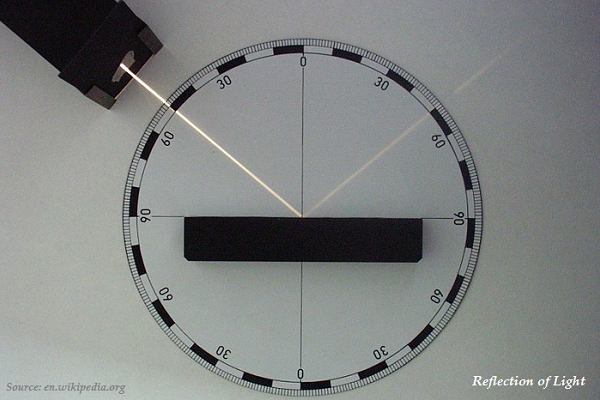• Reflection of light is either specular (just like mirror) or diffuse (retaining the energy.

## Laws of Reflection of Light

• Following are the significant laws of reflection −

• The angle of incidence is equal to the angle of reflection, and

• The incident ray, the normal to the mirror at the point of incidence and the reflected ray, all lie in the same plane.

• The laws of reflection described above are applicable to all sorts of reflecting surfaces including spherical surfaces.

• Image formed by a plane mirror is always virtual and erect.

## Spherical Mirror

• The spherical mirror, whose reflecting surface is curved inwards (as shown in the image given below), i.e. face towards the center of the sphere, is known as concave mirror.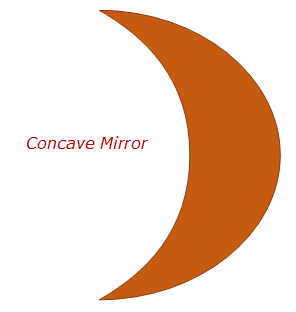• The spherical mirror, whose reflecting surface is curved outwards (as shown in the image given below), is known as a convex mirror.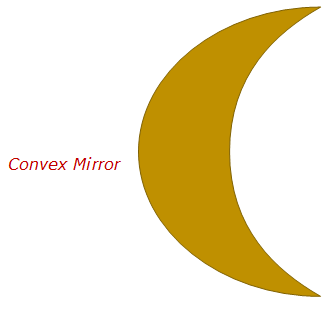• The center of the reflecting surface of a spherical mirror is a point known as pole represented by English letter ‘P.’

• The reflecting surface of a spherical mirror forms a part of a sphere, which has a center, known as the center of curvature represented by English letter ‘C.’

• Remember, the center of curvature is not a part of the mirror, but rather it lies outside the reflecting surface.

• In case of concave mirror, the center of curvature lies in front of it.

• In case of convex mirror, the center of curvature lies behind the mirror.

• The radius of the sphere of which, the reflecting surface of a spherical mirror forms a part, is known as the radius of curvature of the mirror and represented by the English letter ‘R.’

• Remember, the distance pole (P) and center of curvature (C) is equal to the radius of curvature.

• The imaginary straight line, passing through the pole and the center of curvature of a spherical mirror, is known as the principal axis (see the image given below).

• All the reflecting rays meeting/intersecting at a point on the principal axis of the mirror; this point is known as principal focus of the concave mirror. It is represented by English letter ‘F’ (see the image given below).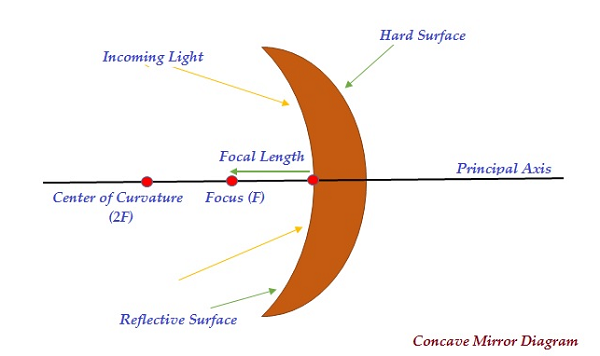• On the other hand, in case of convex mirror, the reflected rays appear to come from a point on the principal axis, known as the principal focus (F) (see the image given below).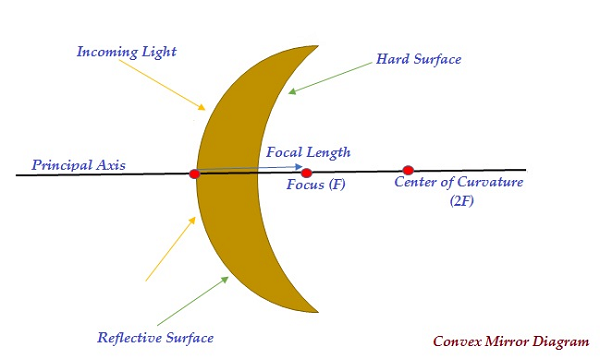• The distance between the pole (P) and the principal focus (F) of a spherical mirror is known as the focal length and it is represented by the English letter ‘f’ (see the image given above).

• The diameter of the reflecting surface of spherical mirror is known as its aperture.